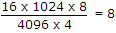# Electronics and Communication Engineering - Digital Electronics - Discussion

44.

A memory system of size 16 k bytes is to be designed using memory chips which have 12 address lines and 4 data lines each. The number of such chips required to design the memory system is

 [A]. 2 [B]. 4 [C]. 8 [D]. 18

Explanation:.

 Anilkumar said: (Jun 3, 2014) What is the exact formula used?

 Athi said: (Jun 18, 2014) How did the answer come?

 Daleep said: (Aug 3, 2014) Logic behind this calculation?

 Krishna said: (Jan 15, 2015) 16K bytes = 16*1024*8. 12 address lines = 2^12 bytes. 4 data lines. Now the simplification is direct.

 Sai said: (Aug 19, 2015) 16k means 16*1000 but you used 1024 why?

 Jayasankar said: (Dec 9, 2015) 16k means 16*1024 but how 8 will comes?

 Shikha said: (Feb 6, 2016) Because in digital byte means 8. = 2^10 = 1026 is called kilo.

 Kumar said: (Aug 4, 2016) 2^16 = 2^10 * 2^6. 1024 * 64 BYTE. 1024 * 64 * 8 BIT.

 Preethi said: (Aug 30, 2016) The numerator is in bits and denominator is in bytes. How could it be possible?

 Dharwendra Pandit said: (Mar 22, 2017) 1 kilobyte = 1024 byte. 1 byte = 8 bit. So 16 * 1024 * 8 bit.

 Aswathy said: (Mar 25, 2017) Number of chips required= Required size/given size. Required size = 16 Kb 1kb = 1024 bytes 1 byte = 8 bits therefore 16 KB = 16 * 1024 * 8 bits. Now, we have 12 address lines and 4 data lines, 12 address lines means 2^12 bytes which is equal to 4096 bytes. Generally, 1024 * 8 means that 1024 locations are there and each location can store 8 bits. 1024 * 2 means that 1024 locations are there and each location can store 2 bits. Data lines means no of bits. Therefore as per the question given above only 4 data lines are there which means only 4 bits can be stored. So, 4094 * 4 indicates 4096 location are there and each location can store 4 bits. therefore the answer to the question is 16*1024*8bits/4096*4bits

 Twinkle said: (May 11, 2017) Thank you @Aswathy

 Kapil said: (Sep 3, 2017) With the help of 12 address lines, we can create 2^12 data locations. Each data location has 4 bits. So the size of memory in each chip = no. of data locations * size of each data location = 2^12 * 4 bits. The required memory system is 16KB i.e. 16 kilo bytes = 16 * 1024 * 8 bits. The Number of chips required = Total memory required / memory of each chip. = 16 * 1024 * 8 bits / 2^12 * 4 bits = 8 chips.

 Guru said: (Aug 3, 2019) Thank you @Kapil.

 Manivannan said: (May 5, 2020) Thank you @Aswathy.

 Sridevi said: (May 7, 2020) Hello all, The memory system size to be designed is 16kb in total. Given memory, chip specs is 12 address line and 4 data lines. Ques: How many memory chips with given specs is required to design a 16 kb system? Ans: No.of mem chips = System size/ given specs size. = 16 * 1024 * 8 bits / 2^12 * 4 bits. Numerator: 16 kb can be written as 16* (1k= 1024)*(1byte= 8 bits) Denominator: (address lines in powers of 2)*(4-> Data lines refer to no of bits). Final answer= 8 chips.

 Aaryan said: (Jul 26, 2020) Required Total Size of memory = 16KByte = 16 *1024 *8 = 2^17 Available memory chip with 12 address line and 4 data lines so available memory size = [2^12] *4 = 2^14. No of chips required = required total size of memory/available chip size = [2^17]/[2^14] = 2^3 = 8.

 Deepa said: (Sep 16, 2021) Thank you @Aswathy.

 Deepa said: (Sep 16, 2021) Thank you @Aswathy.